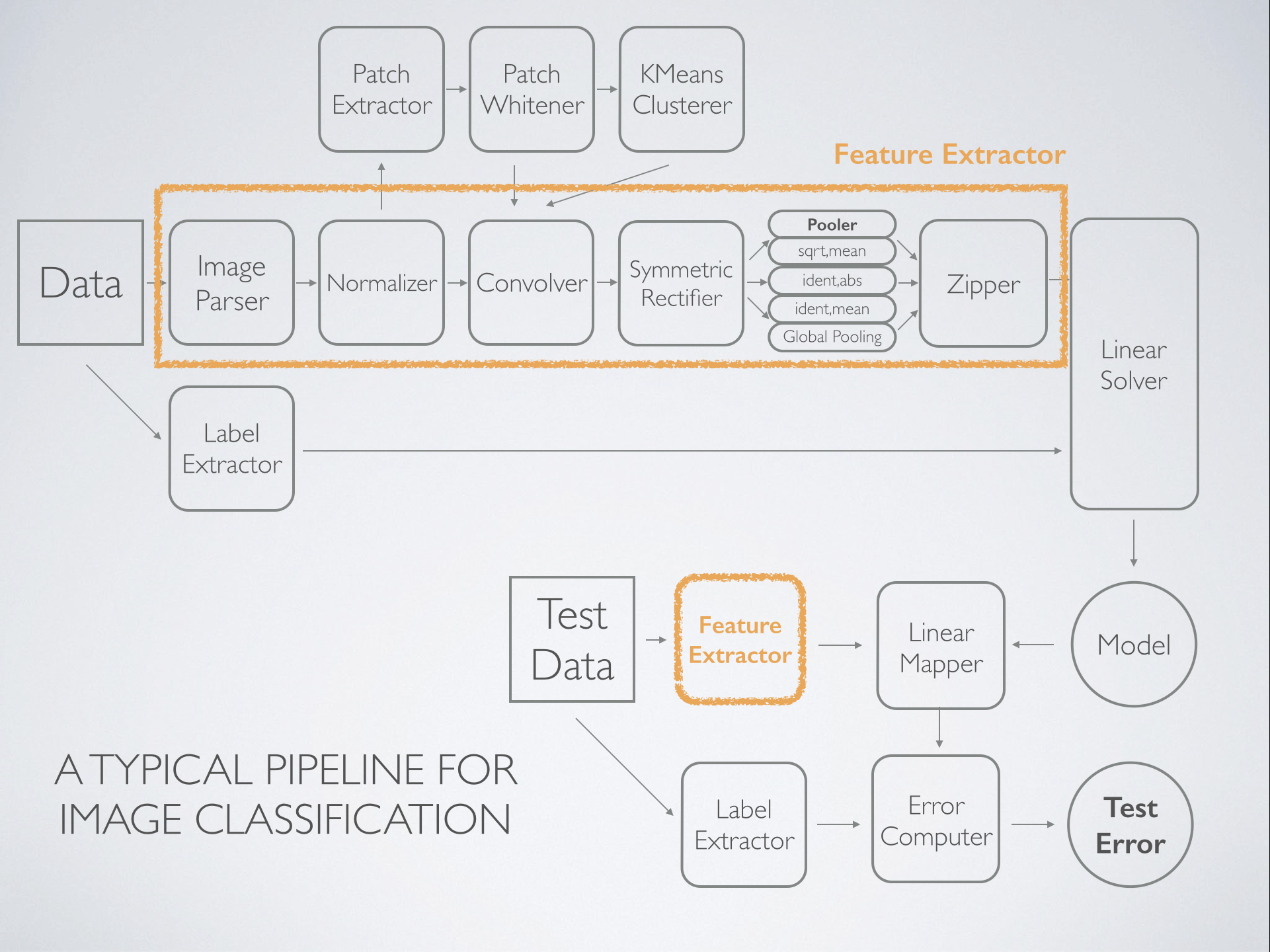(Note) Contents in bold are included in Coursera Machine Learning lectures. A few topics are not identified---regularized regression, neural networks, and anomaly detection.

• Feature extraction and transformation
• Basic statistics: summary statistics, correlations, hypothesis testing
• Anomaly detection: k-NN (k-Nearest Neighbors)
• Neural networks: perceptron, convolutional neural network
• Optimization: stochastic gradient descent, limited-memory BFGS (L-BFGS, Broyden–Fletcher–Goldfarb–Shanno)

Figure: machine learning algorithm maps: (A) scikit-learn; (B) dlib.

## Problems

Learning problems can be roughly categorized as either supervised or unsupervised. Supervised learning builds a statistical model to predict or estimate an output (label) based on some inputs (features): classification if label is categorical, regression if label is quantitative. Unsupervised learning describes the relationships and structure among a set of inputs: dimensionality reduction, clustering.

Other areas of machine learning: Reinforcement learning is concerned with maximizing the reward of a given agent (person, business, etc).

### Regression

linear regression

### Classification

• Linear classifiers:
• Generative model: linear discriminant analysis (LDA), naive Bayes classifier;
• Discriminative model: Logistic regression (logit), support vector machines (SVM), perceptron;
• Isotonic regression;

### Clustering

• k-means clustering;
• hierarchical clustering (dendrogram);
• Gaussian mixture;
• power iteration clustering (PIC);
• latent Dirichlet allocation (LDA);

Standardization is required in case of different units.

### Dimensionality Reduction.

• Principal component analysis (PCA): find the (orthogonal) directions in a Euclidean space that successively explain the most sample variance (minimize the residual sum of squares);
• Singular value decomposition (SVD);## Programming Tools

C++/CUDA:

JVM (Java, Scala):

• H2O: generalized linear models, gradient boosting machine (also supports random forest), generalized lower rank models, deep neural network;
• Spark: MLlib (not nearly as good);
• Deeplearning4j;

R: glmnet, randomForest , gbm, e1071 (interface to libsvm), caret, and more;

Python: scikit-learn sklearn; Pylearn2, Theano;

Benchmark for GLM, RF, GBM: For the algorithms it supports, H2O is the fastest and as accurate on data over 10M records that fit in memory of a single machine. Benchmark for GBM